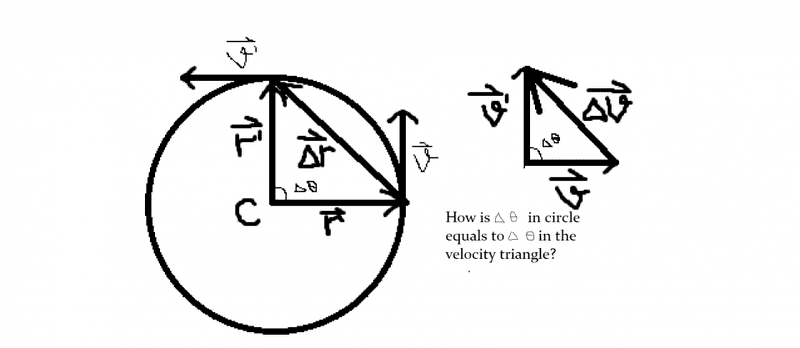# Uniform circular motion?

Member advised to use the homework template for posts in the homework sections of PF.How is Δθ in circle equals to angle in velocity vectors triangle?
I tried using simple geometry but I can't.

Doc Al
Mentor
Try this:

What if r' were right on top of r? What is the angle between them? What is the angle between the velocity vectors?

Now move r' just a bit, an angle Δθ from r. How does the angle between the velocity vectors change?

•Hardikph
Delta2
Homework Helper
Gold Member
Its because they are angles that have sides vertical to each other. r' is vertical to v' and r is vertical to v so the angle of (r',r) equals the angle of (v',v).

•Hardikph
Delta2 and Clara I appreciate your explaination but can you please describe some '90 degree usage'.

Doc Al
Mentor
Another way to look at it: Realize that the velocity vectors are always 90° rotated compared to the r vectors. So if the angle that an r vector makes with the x-axis is θ, then the corresponding velocity vector must make an angle of θ + 90°.

Delta2 and Clara I appreciate your explaination but can you please describe some '90 degree usage'.

In the photo, θ+a = 90..(1) , a+b=90...(2), (1)-(2), θ=b , so it proves your statement "Δθ in circle equals to angle in velocity vectors triangle".
I hope it helps.

•Delta2
James R
•